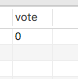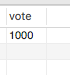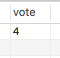## 数据库并发处理

``\$ ab -c 100 -n 1000 http://127.0.0.1/a.php``

`-c 100 -n 1000` 表示一共有1000个请求，每次并发请求100个。

## MysqlMysql的update语句可以保证并发数据的一致性,代码如下:

``mysql_query('UPDATE table1 SET vote=vote+1 WHERE id=1',\$con);``

``\$ ab -c 100 -n 1000 http://127.0.0.1/a.php````````\$res=mysql_query('SELECT vote FROM table1 WHERE id=1',\$con);
\$res=mysql_fetch_array(\$res);
mysql_query('update table1 set vote='.\$res['vote'].'+1 where id=1',\$con);```````SELECT * FROM parent WHERE NAME = 'Jones' LOCK IN SHARE MODE;`

``````SELECT counter_field FROM child_codes FOR UPDATE;
UPDATE child_codes SET counter_field = counter_field + 1;``````

``````mysql_query("SET AUTOCOMMIT=0",\$con);
mysql_query('BEGIN',\$con);

\$res=mysql_query('SELECT vote FROM table1 WHERE id=1 FOR UPDATE',\$con);
\$res=mysql_fetch_array(\$res);
mysql_query('UPDATE table1 SET vote="'.(\$res['vote']+1).'" WHERE id=1',\$con);

mysql_query('COMMIT',\$con);````````````mysql_query("SET AUTOCOMMIT=0",\$con);
mysql_query('BEGIN',\$con);

mysql_query('LOCK TABLES table1 WRITE',\$con);

\$res=mysql_query('SELECT vote FROM table1 WHERE id=1',\$con);
\$res=mysql_fetch_array(\$res);
mysql_query('UPDATE table1 SET vote="'.(\$res['vote']+1).'" WHERE id=1',\$con);

mysql_query('UNLOCK TABLES',\$con);

mysql_query('COMMIT',\$con);
``````